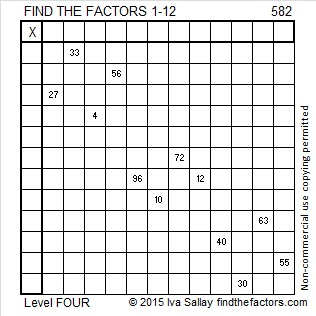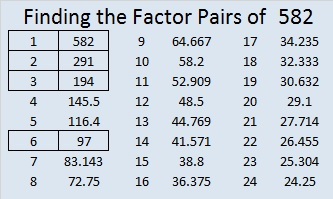# 582 and Level 4

582 is the sum of all the prime numbers from 59 to 89. Can you list those eight consecutive primes?

582 is also the hypotenuse of the Pythagorean triple 390-432-582. Which of 582’s factors is the greatest common factor of those three numbers?Print the puzzles or type the solution on this excel file: 12 Factors 2015-08-10

—————————————————————————————————

• 582 is a composite number.
• Prime factorization: 582 = 2 x 3 x 97
• The exponents in the prime factorization are 1, 1, and 1. Adding one to each and multiplying we get (1 + 1)(1 + 1)(1 + 1) = 2 x 2 x 2 = 8. Therefore 582 has exactly 8 factors.
• Factors of 582: 1, 2, 3, 6, 97, 194, 291, 582
• Factor pairs: 582 = 1 x 582, 2 x 291, 3 x 194, or 6 x 97
• 582 has no square factors that allow its square root to be simplified. √582 ≈ 24.124676—————————————————————————————————This site uses Akismet to reduce spam. Learn how your comment data is processed.